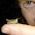## Thursday, July 11, 2013

### The Geiger Counter problem

I am supposed to turn in the manuscript for Think Bayes next week, but I couldn't resist adding a new chapter.  I was adding a new exercise, based on an example from Tom Campbell-Ricketts, author of the Maximum Entropy blog. He got the idea from E. T. Jaynes, author of the classic Probability Theory: The Logic of Science.  Here's my paraphrase
Suppose that a radioactive source emits particles toward a Geiger counter at an average rate of r particles per second, but the counter only registers a fraction, f, of the particles that hit it. If f is 10% and the counter registers 15 particles in a one second interval, what is the posterior distribution of n, the actual number of particles that hit the counter, and r, the average rate particles are emitted?
I decided to write a solution, and then I liked it so much I decided to make it a chapter.  You can read the new chapter here.  And then you can take on the exercise at the end:

This exercise is also inspired by an example in Jaynes, Probability TheorySuppose you buy mosquito trap that is supposed to reduce the population of mosquitoes near your house. Each week, you empty the trap and count the number of mosquitoes captured. After the first week, you count 30 mosquitoes. After the second week, you count 20 mosquitoes. Estimate the percentage change in the number of mosquitoes in your yard.
To answer this question, you have to make some modeling decisions. Here are some suggestions:
• Suppose that each week a large number of mosquitos, N, is bred in a wetland near your home.
• During the week, some fraction of them, f1, wander into your yard, and of those some fraction, f2, are caught in the trap.
• Your solution should take into account your prior belief about how much N is likely to change from one week to the next. You can do that by adding a third level to the hierarchy to model the percent change in N.
I end the chapter with this observation:

The Geiger Counter problem demonstrates the connection between causation and hierarchical modeling. In the example, the emission rate r has a causal effect on the number of particles, n, which has a causal effect on the particle count, k
The hierarchical model reflects the structure of the system, with causes at the top and effects at the bottom.
1. At the top level, we start with a range of hypothetical values for r
2. For each value of r, we have a range of values for n, and the prior distribution of depends on r.
3. When we update the model, we go bottom-up. We compute a posterior distribution of for each value of r, then compute the posterior distribution of r.
So causal information flows down the hierarchy, and inference flows up.

Finally, here's Tom's analysis of the same problem.

#### 1 comment:

1.Thanks Allen, I feel honored! Of course, the real honor belongs to Jaynes.

I didn't remember the example from Jaynes that inspired the mosquito trap, but I must have read it, because I used exactly the same analogy in the title of that post. Damn, I thought I was being original!